Trial ends in

A subscription to JoVE is required to view this content.
You will only be able to see the first 20 seconds.

Torque
Friction
Science Education (Physics I)
Conservation of Momentum

Torque

Overview

Source: Nicholas Timmons, Asantha Cooray, PhD, Department of Physics & Astronomy, School of Physical Sciences, University of California, Irvine, CA

The goal of this experiment is to understand the components of torque and to balance multiple torques in a system to achieve equilibrium. Much like how a force causes linear acceleration, torque is a force that causes a rotational acceleration. It is defined as the product of a force and the distance of the force from the axis of rotation. If the sum of the torques on a system is equal to zero, the system will not have any angular acceleration.

Principles

Torque is defined as the cross product of the distance, r, from the axis of rotation at which a force is applied, and the force, F: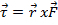, (Equation 1)

where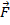is the applied force andis the distance to the axis of rotation. Torque has units of force multiplied by distance and so is measured in Newton meters. Because torque is a vector, it has both magnitude and direction. The direction of the torque is perpendicular to the plane made by the force and distance components. The direction can be determined using one's right hand. Extend the pointer finger in the direction of the first component. Extend the middle finger in the direction of the second component. Once this is done, the direction of the extended thumb is the direction of the torque. An example is a wrench tightening a bolt. A force is applied at the end of the wrench, some distance from the bolt, which provides a torque to rotate the bolt into place. The longer the distance, the larger the torque, as can be seen from Equation 1. The force needed to rotate an object can be reduced significantly by simply increasing the length of the force to the axis of rotation.

A torque on a system will cause an angular acceleration on that system: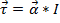. (Equation 2)

Here,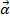is angular acceleration andis the moment of inertia for that system. This is the rotational equivalent of Newton's second law,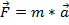, with mass replaced with the moment of inertia and acceleration replaced with angular acceleration.

This experiment will include a meter stick that is able to rotate freely about its axis, as shown in Figure 1.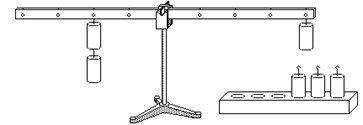Figure 1: Experimental setup.
Weights are attached at various distances from the axis of rotation, which will cause a torque on the system. If the torques on both sides are balanced, the meter stick should not rotate from rest. To examine the torque from a weight or combination of weights, a force scale can be attached to the other side. The force the scale reads multiplied by the distance from the scale to the axis of rotation will be equal to the torque from the weights.

Procedure

1. Using two weights to balance the beam.

1. Start by connecting one 200-g weight to the first hook on the right. Then, connect a 200-g weight to the first hole on the left. If released from rest, the beam should not rotate.
2. Remove the 200-g weight from the left side. Determine using Equation 1 where a 100-g weight would need to be placed to balance the torque from the right side. Place the weight and confirm the prediction.

2. Using three weights to balance the beam.

1. Connect a 100-g weight to the first hook on the right. Place a 100-g weight on the third hook to the right.
2. Determine where to place a 200-g weight on the left side to balance the torques.
3. Determine where to place a 100-g weight on the left side to balance the torques.

3. Using multiple weights to balance the beam.

1. Connect a 200-g weight to the fourth hook on the right side.
2. Using any combination of 100-g and 200-g weights find three ways in which the torque from the right side can be balanced on the left side.
3. With the 200-g weight still connected to the fourth hook on the right side, connect a force scale to the first hook on the left side and pull until it is in equilibrium. Make sure to keep the force scale perpendicular to the beam. Record the force. Do this for each hook on the left side and record the values.
4. With the 200-g weight still connected to the fourth hook on the right side, use a protractor to rotate the beam 30°. Attach the force scale to the third hook on the left and record the force. Repeat for 60°.

Torque is the underlying force that governs rotation and is useful for describing the operation of both simple and complex machines.

Much like how a net force causes linear acceleration a in a translational system, a net torque, typically represented by the Greek letter t, is a force that causes angular acceleration a in a rotational system.

However, if multiple torques acting on a system are made to balance one another, then the net torque will be zero, and the system will be in equilibrium.

The goal of this video is to understand the components of torque by placing weights at different positions on a freely rotating beam to achieve rotational equilibrium.

Before using weights to balance a beam, let's revisit the concepts of torque and rotational equilibrium. A good example of torque is when you have a flat tire and have to use a wrench to loosen a nut before you can change it.

Torque is defined as the cross product of the distance, r, from the axis of rotation at which the force is applied and the force. This distance is also called the lever arm. Note that only the perpendicular component of the force, found using the sin of the angle theta between the force and the rotation arm, contributes to the magnitude of the torque.

It is evident from the equation that by moving the applied force from the middle of the wrench to the end, you double the lever arm and thus double the torque being used to loosen the nut. If the nut still won't budge, you might need to figure out how to increase the perpendicular force.

Now consider another system, where a weight of mass m is attached to a beam that can rotate. Knowing the relationship between linear and angular acceleration, and multiplying both sides of the equation by r, gives a new definition for torque. Now, mr2 is nothing but the system's rotational inertia I and this equation of torque represents the rotational equivalent of Newton's second law, where a torque can cause angular acceleration. Please watch JoVE's Science Education video on Rotational Inertia for further information on this subject.

Now, if the beam is leveled and the weight is removed, there is no net torque on the system and so the angular acceleration must also be zero. Therefore, the system at rest will not rotate and is said to be in rotational equilibrium. For more information on this concept, please watch the video on Equilibrium and Free-Body Diagrams.

Rotation equilibrium can also be established by properly positioning weights on opposite sides of the axis of rotation, so that they equally oppose one another. Conventionally, with respect to the axis of rotation, torque is positive for counter-clockwise rotation and negative for clockwise rotation.

Now that you understand how torque can affect a rotational system, let's see how to apply these forces to achieve equilibrium. This experiment consists of a beam with equally spaced hooks for attaching weights, a protractor, a force scale, and numerous weights with 100 g and 200 g masses.

Initially, two weights are used to establish rotational equilibrium with a 200 g weight connected to the first hook on the right. Connecting another 200 g weight to the first hook on the left should prevent the beam from rotating. Remove the weight from the left side and place a 100 g weight in the proper position to balance the torque from the right side.

Next, three weights are used to balance the torque starting with 100 g weights on both the first and third hooks on the right. Properly position a 200 g weight on the left side so that the net torque on the system is zero. Next, remove the weight and use a 100 g weight to re-establish equilibrium.

Subsequently, multiple weights are employed to balance the beam with a 200 g weight connected to the fourth hook on the right. Using any combination of 100 g and 200 g weights, determine three configurations on the left-hand side that can achieve rotational equilibrium.

Next, with the 200 g weight still connected to the fourth hook on the right, calculate the force required to balance the torque for each of the hooks on the left. Attach the force scale to the first hook on the left, making sure it is perpendicular to the beam, and pull it down until the beam is level and record force value. Repeat this procedure for each hook on the left.

Lastly, with the 200 g weight still attached, connect the force scale to the third hook on the left and level the beam. And, using a protractor, allow the beam to rotate to the right by 30 degrees. Making sure the force scale is perpendicular to the beam, record the force value. Increase the rotation angle to 60 degrees and record this force value.

Each of the balanced beam experiments confirms that a proper configuration of weights can establish equilibrium where the net torque is zero. No net torque implies that no angular acceleration occurs and therefore the beam does not rotate if released from rest. This rotational equilibrium is particularly evident with the six different configurations of 100 and 200 g weights on the left side, which can balance the 200 g weight attached to the right outermost hook.

In the next experiment, the force scale allowed for a more continuous measurement of the torque required for equilibrium. Since the force scale is perpendicular to the beam, just like the weight, the force FL at equilibrium could be calculated using this formula. And this table shows the calculated force for different hooks on the left side with a constant 200g weight on the outmost hook on the right side.

When the beam is rotated from the horizontal by an angle theta, only a component of the gravitational weight, given by this formula, is contributing to the torque. Consequently, the measured force will be less than the value observed for the level beam and will decrease with increasing angle.

The basic principles of torque can be invaluable when trying to understand rotating mechanical systems and how this can translate to linear motion.

A seesaw perfectly demonstrates torque with people generating force on either side of the fulcrum to create rotation. When both sets of people have similar lever arms, the heavier set of people will generate more torque and the other set of people will be lifted up. Conversely, in order to raise the heavier set of people up, they must reduce their moment arm by sliding toward the fulcrum.

A vehicle's torque plays a significant role in its performance, as evident from Newton's second law of angular acceleration. For vehicles with the same inertia, greater torque generates greater angular acceleration, which is directly proportional to the vehicle's linear acceleration. Similarly, if two vehicles have the same acceleration, increased torque would accommodate more inertia and therefore allow a vehicle to tow a massive load.

You've just watched JoVE's introduction to Torque. You should now understand the principles of torque and how it can be used to establish rotational equilibrium or generate angular acceleration. Thanks for watching!

Results

Step 1.2: Connect a 100-g weight to the second hole on the left.

Step 2.2: Connect the 200-g weight to the second hole on the left.

Step 2.3: Connect the 100-g weight to the fourth hole on the left.

Step 3.2: There are six different ways:

1) 200 g - 4th hole

2) 200 g - 1st hole, 200 g - 3rd hole

3) 100 g - 2nd hole, 200 g - 3rd hole

4) 100 g - 1st hole, 200 g - 2nd hole, 100 g - 3rd hole

5) 200 g - 2nd hole, 100 g - 4th hole

6) 100 g - 1st hole, 100 g - 3rd hole, 100 g - 4th hole

Table 1. Results for steps 3.3 and 3.4.

 Hook # Force (N) Force at 30° (N) Force at 60° (N) 1 8 - - 2 4 - - 3 2.7 2.3 1.3 4 2 - -

These results confirm the predictions made by Equation 1. Each weight connected to the beam provides a torque on the system. While weights on one side cause a torque in one direction, weights on the other side cause a torque in the opposite direction. According to Equation 2, when the sum of the torques on the beam is equal to zero, the beam will not rotate when released from rest. In every part of the experiment, when the beam is in equilibrium, the torques must add up to zero.

Applications and Summary

As mentioned earlier, a simple application of torque is using a wrench to tighten a bolt. The important thing to remember is that torque has two components. If it is difficult to tighten a bolt with the wrench in hand, a worker has two options. He can either apply more force or just get a longer wrench. Usually, the latter is the easier choice.

When a car commercial quotes some value of torque, it is a good idea to pay attention. As can be seen by the equation, torque is what makes the wheels on a car accelerate. The more torque, the more acceleration.

A seesaw on the playground is a perfect application of torque. The beam rotates about the fulcrum, and the torque is provided by the people sitting on either end. If one person has more mass, then the torque on that side will be larger and the person on the other side will be lifted up. To get that person down, the person on the ground provides a torque by pushing up with his legs to counter the force of his, weight and he is in turn lifted up.

In this experiment, the two main components of torque were examined. Torque is the product of a force and the distance between the force and an axis of rotation. By placing different weights at different positions on a rotating beam, varying quantities of torque were created. The heavier weight corresponded to a larger force and therefore a larger torque. Placing weights further from the axis of rotation created a larger lever arm, which resulted in a larger torque than if the same weight had been placed closer to the axis of rotation. When the total torque on the beam was equal to zero, the system was in equilibrium.X